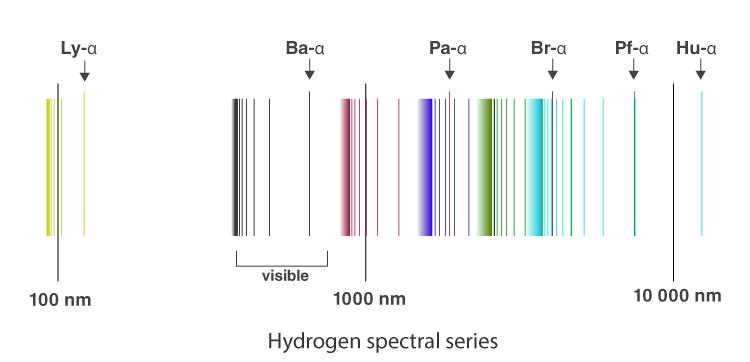# Atomic Spectra

In an atom, electrons have discrete and specific energies. There are more energy states in an atom than there are electrons. When an electron transitions from one energy level to another, it emits light or photon with a specific wavelength.

In any given set of conditions, the collection of all these specific wavelengths is what constitutes the atomic spectrum. Hence, atomic spectra are the spectra of atoms. Here, in this short piece of article, we will be looking at atomic spectra more in detail along with the Rydberg formula and the spectral series of the hydrogen atom.

## What is Atomic Spectra?

Atomic spectra are defined as

The spectrum of the electromagnetic radiation emitted or absorbed by an electron during transitions between different energy level within an atom.

When an electron gets excited from one energy level to another, it either emits or absorbs light of a specific wavelength. The collection of all these specific wavelengths of the atom in a given set of conditions like pressure, temperature, etc is the atomic spectra of atoms. There are three types of atomic spectra and they are emission spectra, absorption spectra, and continuous spectra.

You might want to check the difference between emission and absorption spectra for better understanding.

## Spectral Series of Hydrogen AtomFrom the image above, it is evident that the atomic hydrogen emission spectrum is divided into a number of spectral lines with wavelengths given by the Rydberg formula. The observed spectral lines in the hydrogen emission spectrum are due to the atomic transitions between different energy levels. The spectral series are important in astronomical spectroscopy.

## Rydberg Formula

Using the Rydberg formula, it becomes easy to calculate the spectral lines. Following is the formula:

 $\frac{1}{\lambda }=RZ^{2}(\frac{1}{{n}’^{2}}-\frac{1}{n^{2}})$

Where,

R is the Rydberg constant (1.09737*107 m-1)

Z is the atomic number

n is the upper energy level

n’ is the lower energy level

λ is the wavelength of light

Spectral series of single-electron atoms like hydrogen have Z = 1.

Following is the table for λ in vacuum:

 Series n λ (nm) Lyman series with n’=1 2 121.57 3 102.57 ∞ 91.175 Pfund series with n’=5 6 7460 7 4654 ∞ 2279 Humphreys series with n’=6 7 12.37 8 7.503 ∞ 3.282

Following is the table for λ in the air:

 Series n λ (nm) Balmer series with n’=2 3 656.3 4 486.1 ∞ 364.6 Paschen series or Bohr series with n’=3 4 1875 5 1282 ∞ 820.4 Brackett series with n’=4 5 4051 6 2625 ∞ 1458

## Atomic Spectroscopy

Atomic spectroscopy is the study of the electromagnetic radiation absorbed or emitted by the atoms. There are three types of atomic spectroscopy and they are:

• Atomic emission spectroscopy: This involves the transfer of energy from the ground state to an excited state. The electronic transition can be explained in atomic emission.
• Atomic absorption spectroscopy: For absorption to take place there should be identical energy difference between the lower and higher energy levels. The atomic absorption spectroscopy principle uses the fact that the free electrons generated in an atomizer can absorb radiation at specific frequency. It quantifies the absorption of ground-state atoms in the gaseous state.
• Atomic fluorescence spectroscopy: This is a combination of atomic emission and atomic absorption as it involves radiation of both excitation and de-excitation.

## Uses of Atomic Spectroscopy

• It is used for identifying the spectral lines of materials used in metallurgy.
• It is used in pharmaceutical industries to find the traces of materials used.
• It can be used to study multidimensional elements.

Stay tuned with BYJU’S to know more about other Physics related concepts.

Related Physics Articles: